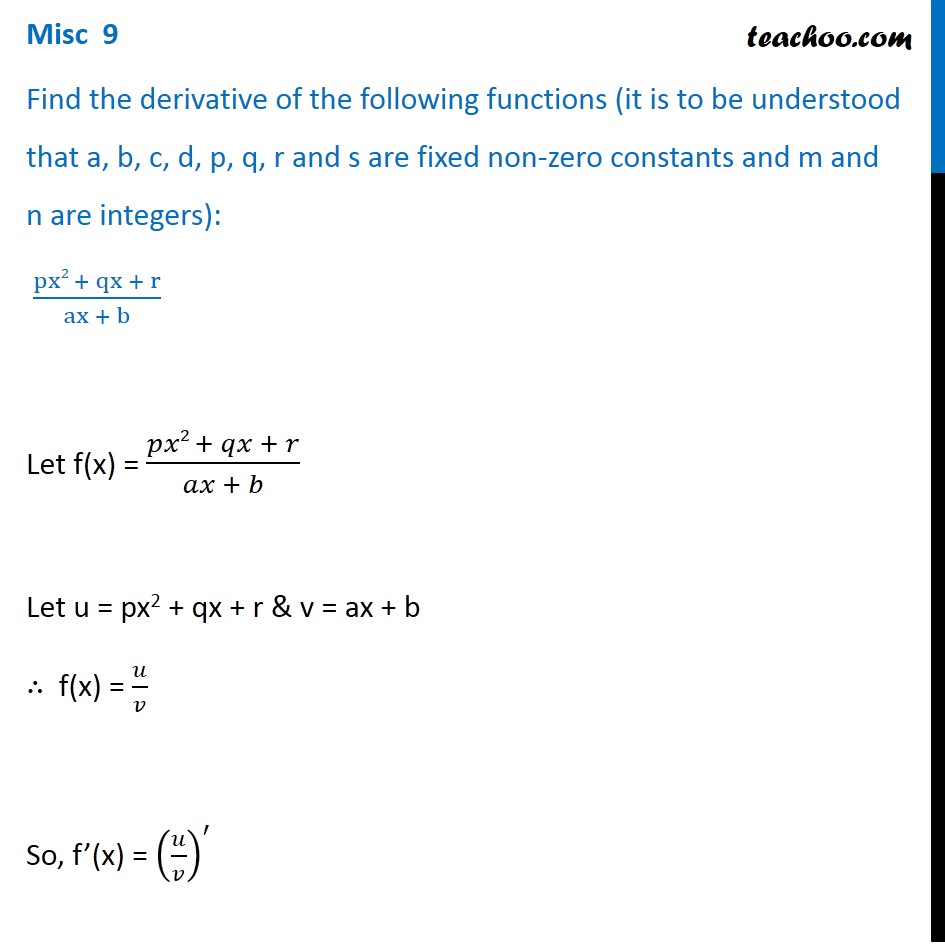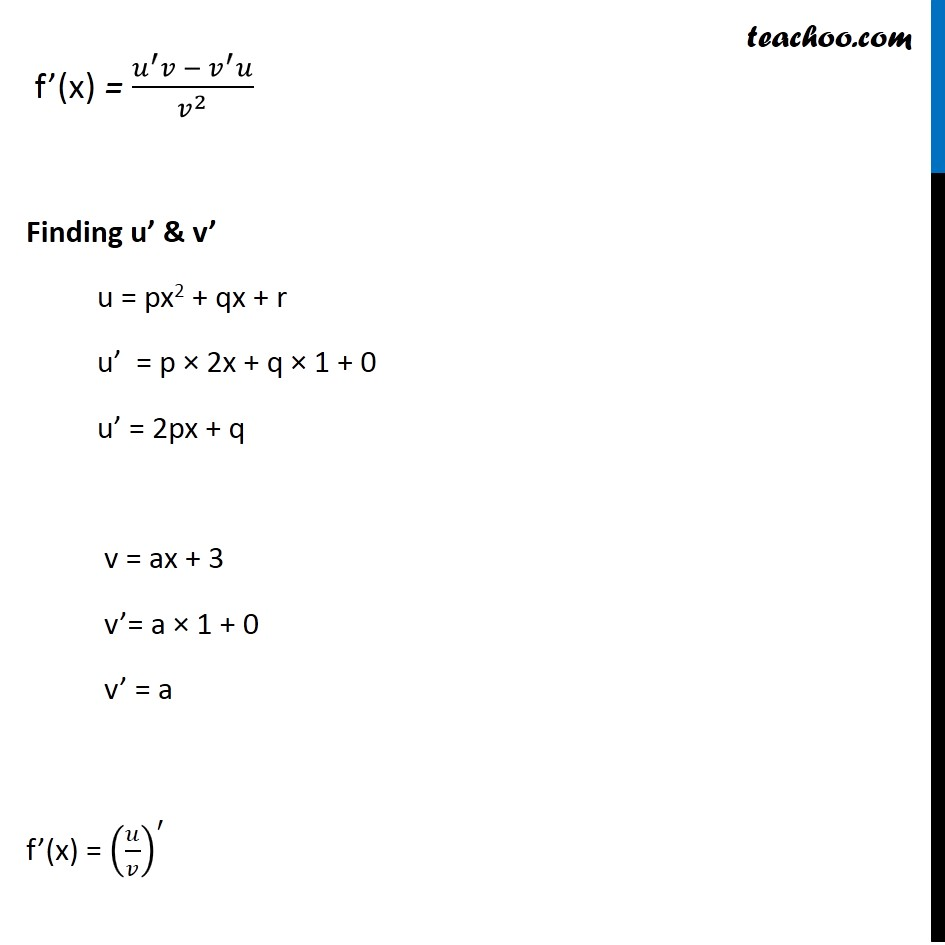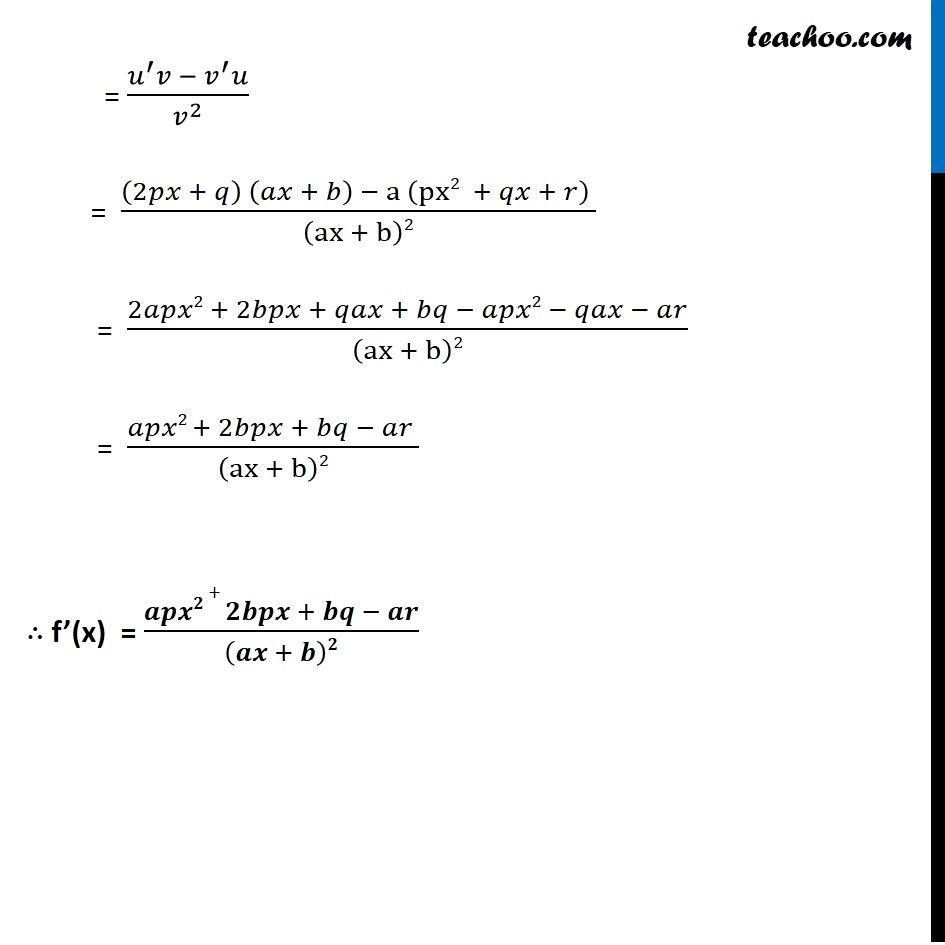Miscellaneous

Chapter 12 Class 11 Limits and Derivatives
Serial order wiseLearn in your speed, with individual attention - Teachoo Maths 1-on-1 Class

### Transcript

Misc 9 Find the derivative of the following functions (it is to be understood that a, b, c, d, p, q, r and s are fixed non-zero constants and m and n are integers): (px2 + qx + r)/(ax + b) Let f(x) = (𝑝𝑥2 + 𝑞𝑥 + 𝑟)/(𝑎𝑥 + 𝑏) Let u = px2 + qx + r & v = ax + b ∴ f(x) = 𝑢/𝑣 So, f’(x) = (𝑢/𝑣)^′ f’(x) = (𝑢^′ 𝑣 − 𝑣^′ 𝑢)/𝑣^2 Finding u’ & v’ u = px2 + qx + r u’ = p × 2x + q × 1 + 0 u’ = 2px + q v = ax + 3 v’= a × 1 + 0 v’ = a f’(x) = (𝑢/𝑣)^′ = (𝑢^′ 𝑣 −〖 𝑣〗^′ 𝑢)/𝑣^2 = ((2𝑝𝑥 + 𝑞) (𝑎𝑥 + 𝑏) − a (px2 + 𝑞𝑥 + 𝑟) )/(ax + b)2 = (2𝑎𝑝𝑥2 + 2𝑏𝑝𝑥 + 𝑞𝑎𝑥 + 𝑏𝑞 − 𝑎𝑝𝑥2 − 𝑞𝑎𝑥 − 𝑎𝑟)/(ax + b)2 = (𝑎𝑝𝑥2 + 2𝑏𝑝𝑥 + 𝑏𝑞 − 𝑎𝑟 )/(ax + b)2 ∴ f’(x) = (𝒂𝒑𝒙𝟐 + 𝟐𝒃𝒑𝒙 + 𝒃𝒒 − 𝒂𝒓)/(𝒂𝒙 + 𝒃)𝟐